# Double Discount Calculator – This app can calculate double discount price easily.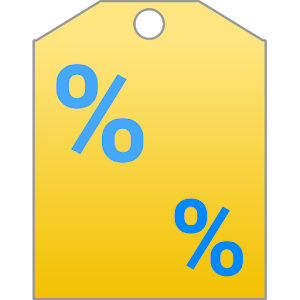This app can calculate double discount price easily.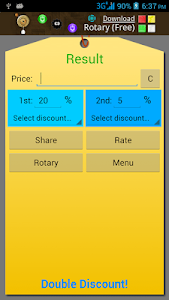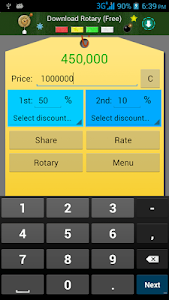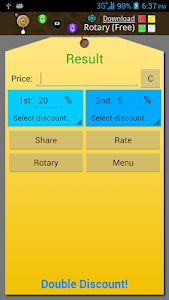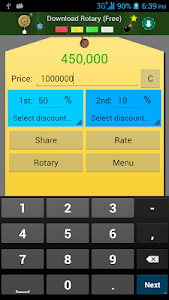This app can calculate double discount price easily. Discount price is calculated by first discount then second discount.

Example:
Item with price 1000000 which have first (1st) discount 50% and second (2nd) discount 10% will be calculated as 450000 (double discount price).
1000000 with 50% = 500000, then 500000 with 10% = 450000

Note:
* The result is rounded to two decimal places.